# How to Add Characters or Strings Before or After Text for Multiple Cells in Excel

Sometimes we often need to add some characters or strings in uniform before or after the text in each cell. If we update cells by copy and paste the characters or strings one by one, it will cost a lot of time, and the work also seems very boring. So, we need to find a simple and convenient way to add these uniform characters/strings. This article will help you to solve your problem now! In this article, we provide three methods, you can read steps of each method, and select one method you like the most. Let’s get started.

See the example below.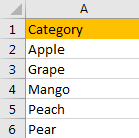If we want to add “Fruit -” before each fruit in A2:A6, how can we do?

## Method 1: Add Characters/Strings for Multiple Cells by Connecter “&”

We can use connecter “&” to adjoin two strings in two adjacent cells.

Step 1: Add “Fruit -“ in cell B2.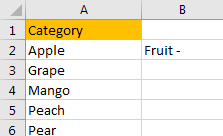Step 2: In cell C3, enter =B2&””&A2.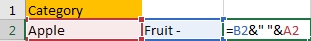Step 3: Click Enter to get the result.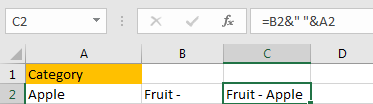Notes:

In step#2, we entered the formula =B2&””&A2, as in cell B2 we entered “Fruit –“ without any blank after “-“, so in the formula we need to add a blank between B2 and A2.

If we entered string “Fruit – “ with a blank after “-“, we only need to enter the formula =B2&A2. See screenshot below: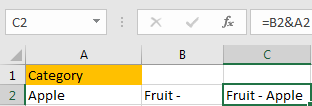After step#3, we can use the same way to fill C3:C6. Just drag B2 to other B cells, and the drag the fill handle down to fill C3:C6.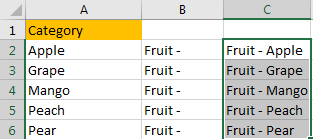If we need to add “- Fruit” at the end of each cell, we can adjust the sequence of the cell.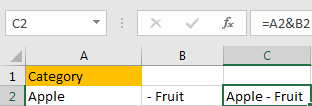## Method 2: Add Characters/Strings for Multiple Cells by Formula CONCATENATE

Step 1: Follow above step#1, in cell C2 enter the formula =CONCATENATE(B2,” “,A2).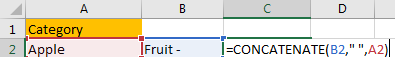Step 2: Click Enter. Verify that we get the same result in above step#3.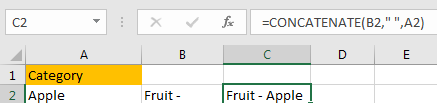Step 3: If we want add strings in B2 after A2, just enter the formula =CONCATENATE(A2,” “,B2).

## Method 3: Add Characters/Strings for Multiple Cells by VBA

If the cells are not adjacent, above methods are not very useful. So we can edit code then run macro to automatically add strings in excel.

Step 1: Click Developer->Visual Basic or Alt+F11 to load Microsoft Visual Basic for Applications.

Step 2: Click Insert->Module to insert module.

Step 3: Edit code in Module window.

```Sub AddFruit()

For Each cell In Selection

cell.Activate

ActiveCell.FormulaR1C1 = "Fruit - " & ActiveCell.Formula

Next cell

End Sub```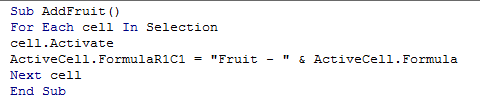Step 4: Save the code and quit Microsoft Visual Basic for Applications.

Step 5: Select on the range A2:A6, then click on Developer->Macro (or Alt+F8) to load Macro.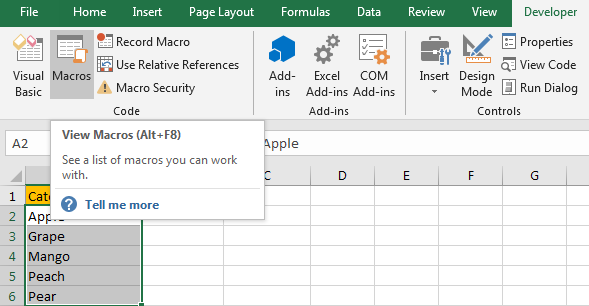Step 6: Previous Macro AddFruit is displayed. Click on the Macro, click Run.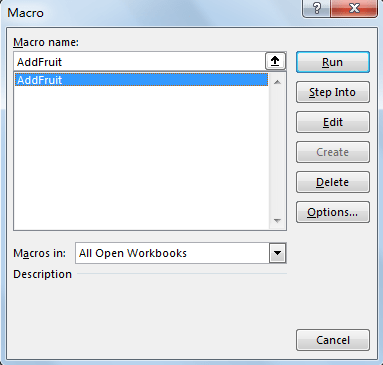Step 7: Verify that “Fruit – “ is added for each cell properly.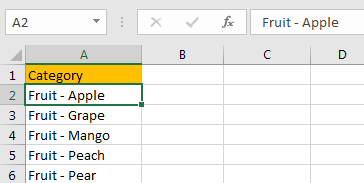Note:

If you want to add “ – Fruit” at the end of each text in cells, you can enter below code:

```Sub AddFruit()

For Each cell In Selection

cell.Activate

ActiveCell.FormulaR1C1 = ActiveCell.Formula & " - Fruit"

Next cell

End Sub```

See screenshot below: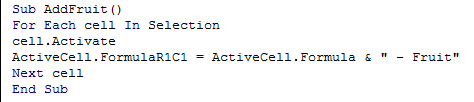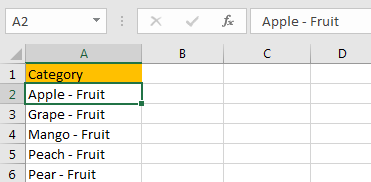Related Posts

Find and Replace Multiple Values

This post will guide you how to find and replace multiple values at once with VBA macro or using formula  in Excel. How do I make multiple find and replace in Excel. Suppose that you have a few cells containing ...

VBA Macro For VLOOKUP From Another Sheet

In the previous post, you should know that how to fix or remove the #N/A error when using VLOOKUP formula to lookup value from another sheet. And this post will show you how to use VBA code to vlookup data ...

How To Insert Comments in Protected Worksheet in Excel

This post will show you how to allow comments in a protected worksheet in Excel. You can easily to insert comments into cells in a normal worksheet in Excel, but if want to insert a comment in a worksheet that ...

How To Convert Text to Upper Cases(Using VBA) in Excel

This post will show you how to switch from lower case to upper case in Excel. and I am going to show you two different ways of converting text to upper cases using formula or VBA macro in Excel 2013,Excel ...

How To Hide Every Other Row in Excel (Using VBA)

This post will show you how to hide alternate rows or columns in Excel or how to hide every third, fourth, fifth row or column in Excel. If you want to hide every other row in your current worksheet, how ...

How to Disable the Save As Prompt in Excel

This post will show you how to use a VBA Macro to save an Excel file and overwrite any existing file without a prompt so that you are going to get the little window that says file already exists do ...

How to Count Cells that Contain even or odd numbers in Excel

This post will guide you how to count the number of cells that contain odd or even numbers within a range of cells using a formula in Excel 2013/2016.How do I count cells that contain odd numbers through the use ...

How to Count Cells that Contain negative Numbers in Excel

This post will guide you how to count the number of cells that contain negative numbers within a range of cells using a formula in Excel 2013/2016.You can count the number of negative numbers in your data using easy functions ...

How to Count Cells Are Not Blank or Empty in Excel

This post will guide you how to count cells that are not blank or empty in a given range cells using a formula in Excel 2013/2016.How do I count the number of cells that are not blank in a particular ...

How to Count Cells Less Than a Specific Value in Excel

This post will guide you how to count the number of cells less than a particular numeric value in a given range cells using a formula in Excel 2013/2016. How do I count cells that are less than a specific ...

Sidebar